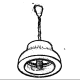# A self optimising moving average

Different markets and different timeframes will need different moving average periods. This article will show a way to construct a self optimising moving average, one which automatically adjusts its period to the charted market and timeframe.

## Reading a simple moving average

I would like to start this new indicator with some thoughts about how to define how “good” a moving average is. Usually my (simplified) standard interpretation of a moving average is, that when it rises and the market trades above the average, I am in bullish mode and would expect the market to rise over the next  bars. This very simple interpretation of a moving average can be quantified, meaning that I can calculate a measure to judge if my assumption is any good. To do so, I just count the number of bars which have been rising and falling while (initial condition) the market has been above the average and the average has been rising. This will give me a number, and let’s say on 52% of the bars in history when the initial condition was met, the market rose on the day after. As the chance is better than 50%, I would conclude that the analysed average is a useful one.

## Self optimising moving average

Instead of just analysing one specific moving average length one could calculate the metrics for all moving averages. The sample implementation (code at the end of the article) will calculate all moving averages within a given parameter range (eg. 5 bars to 200 bars), calculate the winning percentage (rising bars) on the next bar, and then pick the best performing period length.

The moving average on the chart above constantly changes its period to the period, which would have given the best indication in history. As the original criteria has been to maximise the percentage of up-moving bars when the market is above the average and the average is rising, the shown moving average will always be the one which will give you the highest probability of a rising bar (next bar) if the average is rising and the market is trading above it. These periods are shown in green. Otherwise, if the criteria is not met or the winning percentage is below 50 (=no average has a better than 50:50 prediction capability), the indicator is colour coded red.

## Advantage of the perfect average

Beside plotting the best moving average, one could also plot the statistics of the best moving average. This would be the percentage of winning bars when the original criteria is met.

The chart above shows a comparison of 3 different timeframes. A daily, hourly and 5 minute chart. The indicator below shows the edge the best moving average would give when trying to predict rising bars. A level of 5 would mean that you got a 55% chance for a rising bar when selecting the best performing period for your moving average and the average is rising and the market trades above it.

Using the self adjusting average this way, you can easily see in which timeframes or markets a moving average prediction model would be useful. On the chart above you obviously do not want to use this simple indicator interpretation on a 5 minutes chart. If the indicator is colour coded in red, either the market is not trading above the best average, the best average is not rising, or it would give no edge when trying to predict the next bars move.

## Stability of results

As the indicator is based on a statistic over a given period of time, we have to think about stability and outliners. To remove outliner results, the statistics are smoothed. Instead of taking the winning percentage for a given average length, also the 2 results to the left and right are taken into account. The indicator can also plot these smoothed results, so you can see the edge the average prediction would offer with different periods.

On the chart below, the magenta histogram on the right shows the historic edge (smoothed) over the tested periods for the averages. From length 7 to length 148 all results would have been positive (a better than 50:50 chance for a rising bar), the best result was obtained with a period of 31. Observe this with different lengths of history, and you will get a good guess if your market is the right one for this kind of analysis. If the edge is not above 50% with a lot of periods, then this prediction will hardly be useful.

## Link to indicator source code

Find the indicator code over here. The code is written in Equilla, the scripting language of the Tradesignal software.

The indicator uses all available data on the chart to calculate the statistics. You can set the range of periods you want to analyse. If you would like to backtest the indicator (go long when green) then just add the line below at the end of the code (used as strategy)

## 3 thoughts on “A self optimising moving average”

1.Andrei

Hi,

Would you mind me publishing you algorithm of self-optimizing average in Pinescript on TradingView (with appropriate referencing to your article)?

B.R.

•Kahler Philipp

no problem, go on… :-)